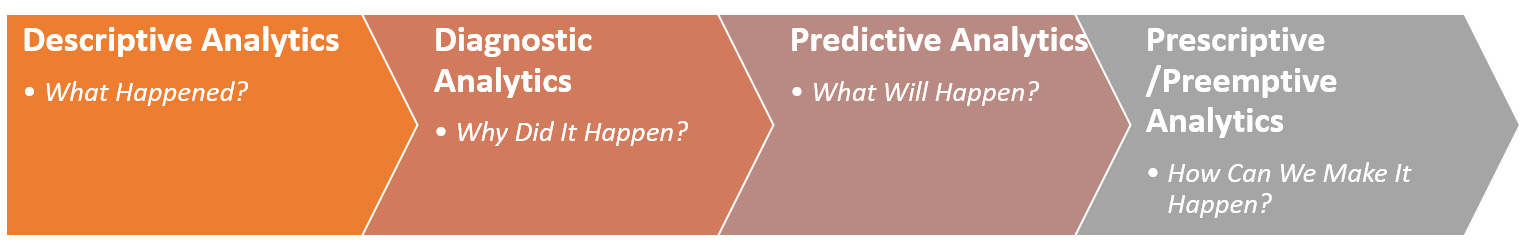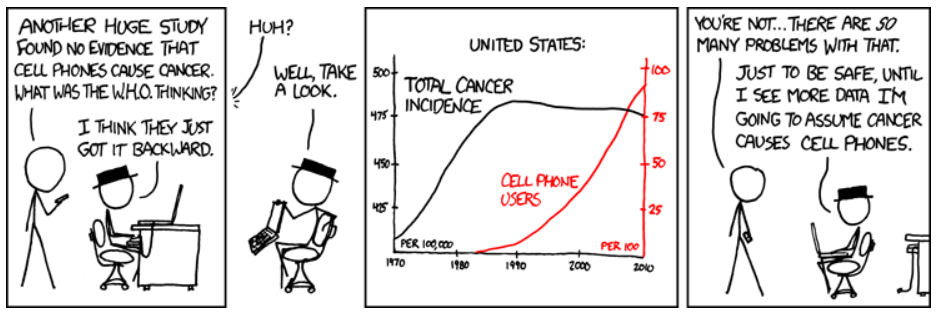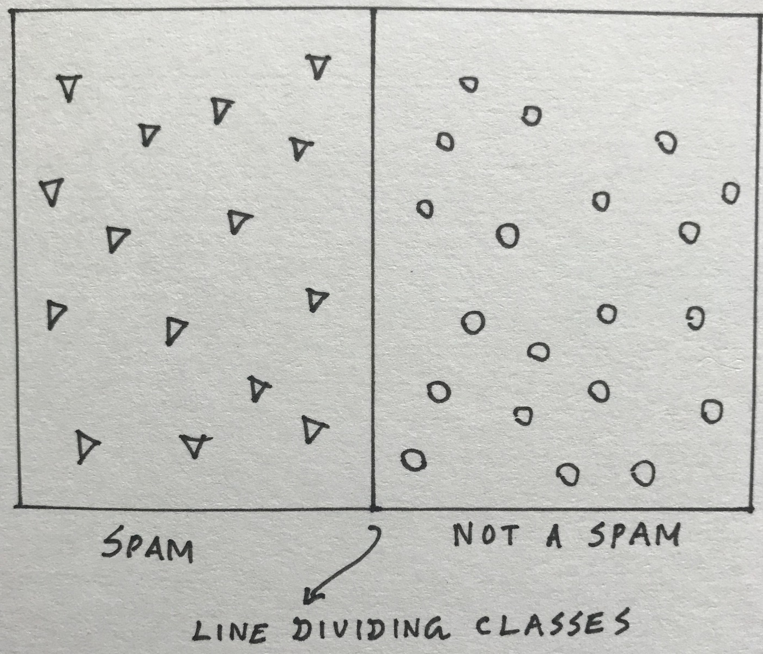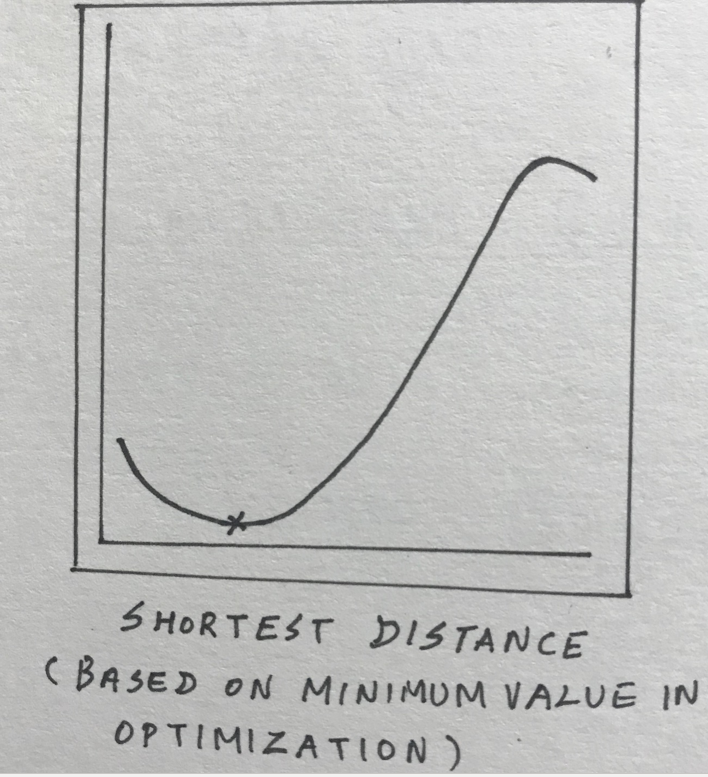{{announcement.body}}
{{announcement.title}}

# Best of Both Worlds: Data Science And Mathematics

DZone 's Guide to

# Best of Both Worlds: Data Science And Mathematics

### Beef up your data science skills.

· Big Data Zone ·
Free Resource

Comment (0)

Save
{{ articles.views | formatCount}} Views
Mathematics is not about numbers, equations, computations, or algorithms: it is about understanding.
~ William Paul Thurston

There are several tools and techniques that don’t require any expertise in Mathematics to solve Data Science problems. However, this article is intended to explore how some Mathematics branches can help to hone scientific and engineering expertise in Data Science, once feature engineering and data preprocessing is done effectively.

Before we move forward, we need to ensure data analysis is done right, since it’s the foundation for solving business problems through Data Science.

A quick recap of different level of analytics:It is not necessary for all business problems to go through every level of analytics. At times, simple descriptive analytics can aid stakeholders in decision-making.

Let us explore how some curriculum of mathematics can help us better understand the field of Data Science.

## Descriptive Analytics

After data preprocessing, it is important to study and interpret data. Statistics come in handy when collecting and analyzing numerical data. While Mathematics and Statistics sound two like different fields, they are not; Statistics is a branch of mathematics dealing with collection, organization, analysis, interpretation, and presentation of data.

Some examples of representing data leveraging descriptive statistics are:

• On average, the weather is around 30 degrees Celsius in Hyderabad during a monsoon. At times, it goes as low as 19 degrees Celsius.
• Exam scores in a Mathematics class range from 60% to 90% with a higher frequency of scores around 70%.
• The number of Income-tax refunds submitted in the financial year (they peak at year-end, so the dataset would most likely have a negative/left skew).

Descriptive statistics offer powerful calculations such as mean, median, mode, deviation, variance, range with which we can derive meaningful summary of data.

## Diagnostic Analytics

While understanding the root causes of an issue may help in predicting business outcomes more efficiently, it’s not always easy to find these causes. Feature engineering can help to narrow down the potential causes.

Correlation analysis helps in identifying the relationship between variables. The below cartoon depicts how correlation and causation are different (source: https://xkcd.com/925/).While correlation doesn’t necessarily justify causation, it certainly helps in identifying relationships and aids in optimization that leads to prescriptive analytics.

Correlation analysis assumes dependency between variables is linear. Linear algebra helps to establish linearity and strength of relationships between variables. In fact, linear algebra plays a critical, role not just in diagnostic analytics, but in text analytics and Artificial Intelligence. Linear algebra operates in multi-dimensional spaces; hence, it is easy to solve any kind of business problem once converted as a mathematical equation.

## Predictive Analytics

This phase is all about predicting future outcomes based on the nature and patterns of data we extracted as part of data analysis.

Forecasting the future with a certain level of reliability with what-if scenarios... sounds like mathematical equations we studied in school, right?

Linear algebra helps in representing problems with equations. Variables and equations can be represented in the form of vectors. Irrespective of the number of variables and equations, we can find solutions to satisfying our constraints.

In classification problems, such as predicting whether a new email is spam, the line is drawn splitting the space of spam and non-spam and placing the new data accordingly.In prediction use cases, such as weather forecasting, it’s all about determining the plane closest to all historical data points (weather from previous days/months/years).## Prescriptive Analytics

Almost all business problems have constraints (time, budget, resources, etc.). Providing our recommendation based on those constraints with a high level of reliability is essential.

Linear programming and linear optimization help in representing complex relationships between variables through linear functions and find optimum points.z

The maxima, minima, gradient descent, and other similar concepts in mathematical optimization come to our rescue in solving complex problems.

Since the prescriptions are based on futuristic events, it’s important to provide recommendations and their quantifiable likelihoods. As a result, probability theory plays a vital role along with optimization techniques.

## Summary

The following branches of Mathematics help in understanding business problems by analyzing data patterns and resolving them with higher reliability.

• Descriptive Statistics: To understand the pattern of data.

• Linear Algebra: To convert business problems into mathematical problems and solve them. In fact, with the power of representing numeric and text data in the form of vectors and metrics, Linear Algebra plays a powerful role in the area of deep learning in Artificial Intelligence as well.

• Linear Programming: To provide the best possible outcomes with given constraints.

• Probability: To provide quantifiable likelihood along with recommendations.

Of course, other branches, such as calculus, play critical roles in deep learning.

In my opinion, adding a flavor of Mathematics in addressing business problems through Data Science provides more options with improved reliability.

I have listed below some of my favorite links to explore mathematics in a more fun way. Happy exploring!

Topics:
data science ,artificial inteligence ,mathematics ,probability and statistics ,linear algebra ,optimization ,prediction ,forecasting ,algorithms

Comment (0)

Save
{{ articles.views | formatCount}} Views

Opinions expressed by DZone contributors are their own.